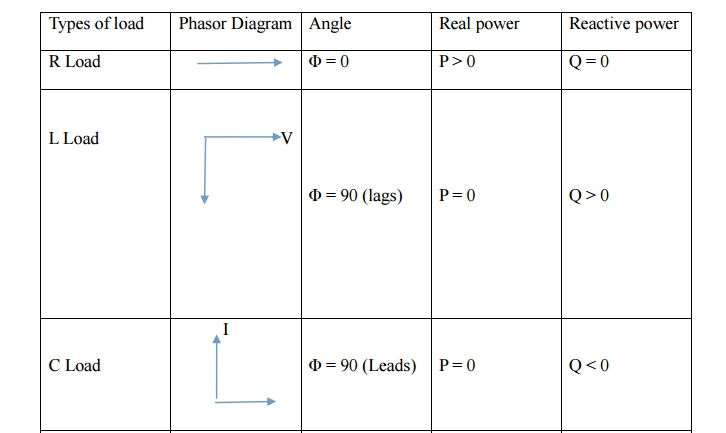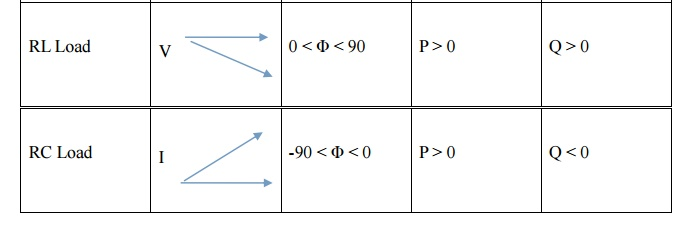Home | | Power System Analysis | Concept of Real and Reactive Power

# Concept of Real and Reactive Power

Let ‘V’ be the Instantaneous voltage, Let ‘i’ be the Instantaneous current

CONCEPT OF REAL AND REACTIVE POWER

Let ‘V’ be the Instantaneous voltage

Let ‘i’ be the Instantaneous current

V = Vm sin ωt

I = im sin (ωt - Φ)

Transmitter power P = V iInductive Load - Absorbs reactive power

Capacitive Load - Generate reactive power

Apparent Power:The product of RMS value of voltage and current

Study Material, Lecturing Notes, Assignment, Reference, Wiki description explanation, brief detail
Power System Analysis : Introduction : Concept of Real and Reactive Power |